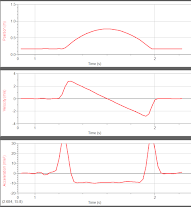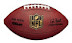Use Google's Chrome browser for proper viewing.

IB Physics SL (2019-2020)

8/15

• Informational Packet / Syllabus
• Introduction to class
• Homework - brainstorm a notation for keeping track of how far an object moves AND in which direction it travels, e.g. a man walks 20 meters eastward, then 20 meters northward, then 10 meters westward; can you write this in a way that the sum of these three motions gives you the man's final position?

8/19

• discuss briefly the history of modeling motion
• differentiate between average and instantaneous speeds and define constant speed
• determine if a toy car moves with constant speed
• create a scatter plot of the motion of a toy car and discuss the significance of its slope
• Homework - read to p. 11 in the booklet (Physics: An Introduction); do the 6 math questions on p. 10

8/21

• define distance, displacement, speed, velocity and acceleration
• "read" position, velocity and acceleration graphs and relate them to one another

8/23

8/27

• derive the kinematic (or suvat) equations
• practice using the kinematic equations
• Homework - solve sample IB test questions on motion graphs; if you have a textbook, read over pp. 27-38 for a review of the material we've covered thus far

8/29

• test over motion graphs, with general questions about distance, displacement, speed (average and instantaneous), velocity and acceleration
• Homework - install Logger Pro on your laptop (on a school-issued laptop, install it from the Software Center; on all other laptops, use these instructions); read pp. 27-38 in your textbook

9/3

• practice interpreting trendlines and using the extracted (velocity and acceleration) information to interpolate and extrapolate (file 1file 2)
• Extra Practice  - match motion to graphs with this interactive.
• Homework - do test corrections on a separate sheet of paper (just rework the missed problems)

9/5

• picture day
• study how objects fall using a "motion detector" - lab instructions
• homework - finish the lab at home; read (and take notes on) pp. 8-16 in your textbook

9/9

• use the kinematic equations for modeling freely falling objects - worksheet
• homework - read about experimental uncertainty (its measurement and propagation in calculations) - handout

9/11

• begin formal lab investigation of free fall acceleration
• the general Lab Report Format

9/13

• calculate lab uncertainties
• the lab report will be due Wed 9/18 (at 11:59 pm) through turnitin.com
• homework - you should get started on the lab report

9/17

• graph the free fall data -- linearize the graph
• homework - complete your lab report; the free fall calculations are due 9/23

9/19

• quiz over free fall  postponed until Monday
• review free fall calculations
• discuss relative motion
• homework - complete free fall calculations (handout)

9/23

• quiz over free fall
• introduction to vectors

9/25

• model 2D projectiles
• homework - complete this worksheet of projectile problems (the answers are given within the document)

9/27

• review projectile problem-solving
• projectile motion lab (online)
• homework - complete the lab
• pay for the IB Physics field trip to iFLY! The trip will be Mon, Oct 21.

10/1

• Mythbusters video
• discuss the effect of air resistance on projectile motion
• solve IB projectile problems
• help with projectiles
• homework - extra projectile practice (key)

10/3

• quiz over projectile motion
• discuss the nature of a "law of nature" and the certainty of our knowledge
• homework - pay for the iFLY field trip!

10/7

10/9

• meeting with counselor
• discuss the concept of a force and the types of forces found within our world (follow this guide and answer questions in your class notebook)

10/11

• review types of forces
• begin lab investigation of Newton's 2nd Law - formal lab info

**No school on Monday or Tuesday**

10/17

• complete the lab
• STUDENTS: before going to iFLY, you or your parent must complete this
• online waiver!! go ahead and do this asap -- reservation #4115004005
• help sheet for adding max and min trend lines to your graph

10/21

• field trip to iFLY !! - some photos of the trip

10/23

• read and process the information on this webpage
• calculate the force of gravity and discuss weightlessness

10/25

• discuss apparent weight
• calculate friction
• draw free-body diagrams and calculate the acceleration of various objects
• homework - the formal lab is due Friday, Oct 25 Saturday, Oct 26 at 11:59 pm on turnitin.com; try to do these problems, after reading the explanations

10/29

• practice drawing free-body diagrams and using F=ma; model an Atwood machine and a modified Atwood machine
• homework - read Unit 2.2 Forces, in your textbook; complete the Forces SL packet
• mini-project - Video yourself asking two individuals (not juniors or seniors in high school) what it means to be weightless and record their responses. Then, in 1 min 30 sec or less, clearly explain what it means to be weightless. Also specifically address any misconceptions offered by the interviewees. Don't say too little; show what you know. Plan what you are going to say before you say it! You do not need to appear in the video throughout, but I should see you at least some and hear you for the entire post-interview section. Props, diagrams and photos can be used, if desired. Upload this video to the linked Google folder by the deadline, 11/6.

11/1

• analyze a block sliding down a ramp (and hanging signs (in translational equilibrium)?)
• discuss Newton's 3rd Law
• homework - if you haven't already, read Unit 2.2 Forces; you can find a bunch of review videos here

11/6

• test over forces (even if you were absent last class)
• the mini-project is due here by class time today; upload it, don't share it; it will count as a major grade

11/8

• define kinetic and potential energy and work

11/12

• derive and use the Work-Kinetic Energy Theorem
• calculate change in kinetic energy from a force-vs-position graph (key)

11/14

• solve problems involving work and energy
• sample problems worked out
• practice solving energy problems (note: I am not assigning the entire packet)
• upcoming: the mousetrap-powered car project will be assigned next week
• upcoming: we will have a test next week on work and energy
• homework - solve at least 12 problems in the green packet [answer key]; complete the four mini-worksheets

11/18

• calculate elastic potential energy
• solve IB test problems involving elastic PE
• homework - complete pages 2 and 3 of the handout (IB problems on EPE)

11/20

• assign the mousetrap-powered car project (due Dec 9) - video help
• solve additional problems involving elastic potential energy

11/22

• test on work and energy

12/3

• discuss the energy test; spend more time learning energy concepts
• carefully read and think through this handout on work and energy
• [we will need to meet during PIT to properly finish this topic; you will need to re-take the exam]
• homework - watch this video on power and efficiency; take notes; we won't discuss the material in class
• re-take the exam Friday before school (8 am sharp) or next Wednesday PIT; your grade will be the higher of the two

12/5

• define momentum, discuss its conservation, and model the transfer of momentum in a 2-body collision
• get help by watching this video

12/9

• the mousetrap-powered cars and videos are due [submit the video here]
• lecture on impulse and its connection to momentum
• homework - complete worksheet of momentum problems

12/11

• solve IB problems on momentum
• calculate impulse from a force-time graph (practice)

12/13

• quiz on momentum
• homework - review for the midterm

12/17

• midterm exam, covering all material from the semester
Lab Report Format

Unit One-Page Overviews

1.1 Measurements in physics

1.2 Uncertainties and errors

1.3 Vectors and scalars

2.1 Motion

2.2 Forces

2.3 Work, Energy, and Power

2.4 Momentum

Unit One-Page Overviews

3.1 Thermal Concepts

3.2 Modelling a gas

6.1 Circular Motion

4.1 Oscillations

4.2 Travelling Waves

4.3 Wave Characteristics

5.1 Electric FieldsFree Fall Motion: s,v,&a graphs

Parabolas in the NFL: the physics of a football puntHow strong is the force of gravity on Earth?